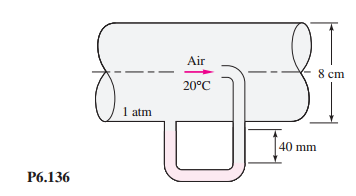### Estimate the centerline velocity

Assignment Help Mechanical Engineering
##### Reference no: EM131147714

For the pitot-static pressure arrangement of Fig. P6.136, the manometer fluid is (colored) water at 20°C. Estimate

(a) the centerline velocity,

(b) the pipe volume flow, and

(c) the (smooth) wall shear stress.#### How much acceleration can fluids endure

How much acceleration can fluids endure? Are fluids like astronauts, who feel that 5g is severe? Perhaps use the flow pattern of Example 4.8, at r= R, to make some estimates

#### What the temperature in the cargo area

How does this compare to the total chilled water cooling capacity, for which the design chilled water temperature difference is ΔTm = 6K? You may assume fully developed flow

#### Find the tower s natural frequency of bending vibration

The antenna tower can be modeled as a steel beam of square section of side 1 in. for estimating its mass and stiffness. Find the tower s natural frequency of bending vibrati

#### What would be the natural period of the system

The natural time period of this system is found to be 0.5 s. If an identical spring is cut so that one part is one-fourth and the other part three-fourths of the original le

#### Find the natural frequency of vibration of the weight

A scissors jack is used to lift a load W. The links of the jack are rigid and the collars can slide freely on the shaft against the springs of stiffnesses k1 and k2 (see Fig

#### Determine the natural frequency of horizontal vibration

A building frame is modeled by four identical steel columns, each of weight w, and a rigid floor of weight W, as shown in Fig. 2.79. The columns are fixed at the ground and

#### Determine the equivalent cross-sectional area of the cables

The time period of axial vibration (in vertical direction) is found to vary from 5 s to 4.0825 s when an additional mass of 5,000 kg is added to the pressure-vessel head. De

#### Find an expression for the natural frequency of oscillation

The inclined manometer, shown in Fig. 2.73, is used to measure pressure. If the total length of mercury in the tube is L, find an expression for the natural frequency of osc

### Write a Review